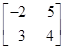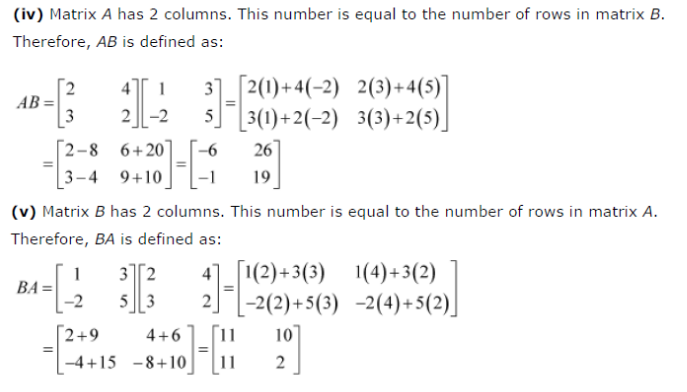# Let A = [ -2 5 3 4 ] B = [ -2 5 3 4 ] c = [ -2 5 3 4 ] . Find each of the following

Let A =B =C =. Find each of the following:
(i) A + B
(ii) A – B
(iii) 3A – C
(iv) AB
(v) BA